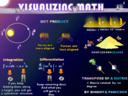# VISUALIZING MATH E-BOOK (WORD FORMAT)(VIA MATH-0-GENIUS YOUTUBE CHANNEL)

\$6BINNOY
0 ratings

Math doesn't exist. Its just a language to understand the underlying physical phenomenon.

What if I tell you that Mathematics is just a language to understand intuitively the following.

UNDERSTAND INTUITIVELY.....

1] How sin( ) is a measure of how much perpendicular two objects/forces are.

2] How cos( ) is a measure of how much parallel two objects/forces are.

3] How in complex numbers

i = rotation by 90 degrees

i^2= rotation by 180 degrees

i^3= rotation by 270 degrees.

i^4 = rotation by 360 degrees.

4] Transpose of a matrix = Rotation by 180 degrees around the diagonal.

5] e^i(angle) = rotation by that angle.

6] sin(30) = 1/2

because at 30 degree the effect of a force reduces to half over the other force/object (as against when the two forces/object are against at 90 degrees w.r.t each other)

7) A X B = |A| |B| sin(angle between A and B).

8) Integration is a shorcut to multiplication which inturn is a shortcut to addition.

And differentiation is a shortcut to division and division inturn is a shortcut to subtration.

9) Log to the base(base = rate of change,say 10) of an effect(say 1000) gives you the cause (3)

It will take 3 days for a bacteria to grow from 1 to 1000 if its growing at a rate of 10 times per day.

https://visualizingmathsandphysics.blogspot.in

SUPPORT PAGE ON PATREON

https://www.patreon.com/BINNOYSPANICKER

And so on.........